# turbo

Turbo colormap array

## Syntax

``c = turbo``
``c = turbo(m)``

## Description

example

````c = turbo` returns the turbo colormap as a three-column array with the same number of rows as the colormap for the current figure. If no figure exists, then the number of rows is equal to the default length of 256. Each row in the array contains the red, green, and blue intensities for a specific color. The intensities are in the range [0,1], and the color scheme is similar to that of the `jet` colormap, but the transitions between colors are more perceptually uniform than in `jet`. The color scheme looks like this image.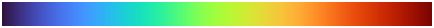```

example

````c = turbo(m)` returns the colormap with `m` colors.```

## Examples

collapse all

Plot a surface and assign the turbo colormap.

```surf(peaks); colormap('turbo');```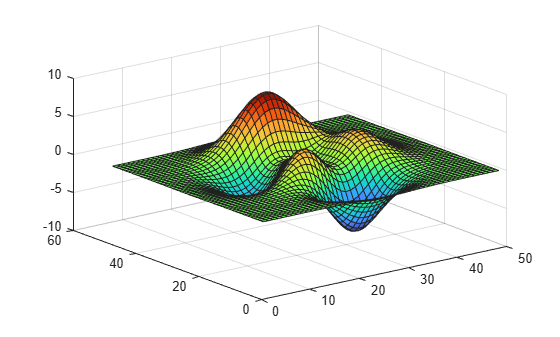Get the turbo colormap array and reverse the order. Then apply the modified colormap to the surface.

```c = turbo; c = flipud(c); colormap(c);```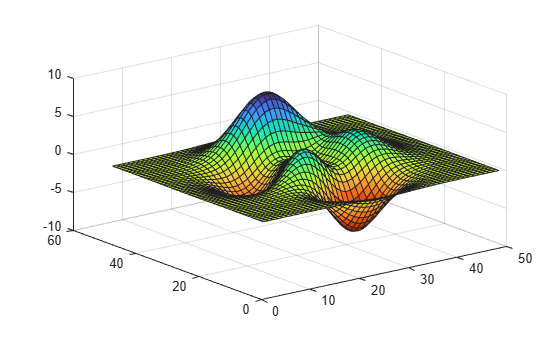Get a downsampled version of the turbo colormap containing only ten colors. Then display the contours of the `peaks` function by applying the colormap and interpolated shading.

```c = turbo(10); surf(peaks); colormap(c); shading interp;```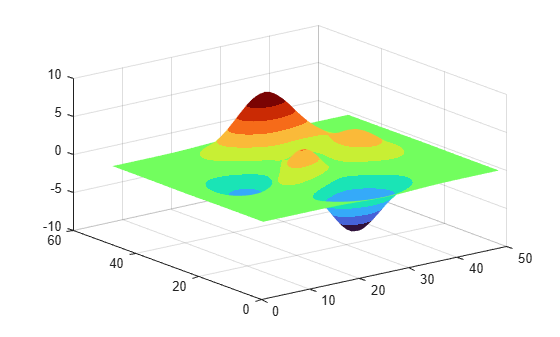Create a tiled chart layout containing two pseudocolor plots of the `peaks` data set. The left plot uses the jet colormap, and the right plot uses the turbo colormap. Notice that the transitions between colors in the turbo plot are less severe than they are in the jet plot.

```t = tiledlayout(1,2); [X,Y,Z] = peaks; % Left image ax1 = nexttile; s1 = pcolor(ax1,X,Y,Z); s1.EdgeColor = 'none'; s1.FaceColor = 'interp'; ax1.Colormap = jet; title(ax1,'Jet'); % Right image ax2 = nexttile; s2 = pcolor(ax2,X,Y,Z); s2.EdgeColor = 'none'; s2.FaceColor = 'interp'; ax2.Colormap = turbo; title(ax2,'Turbo');```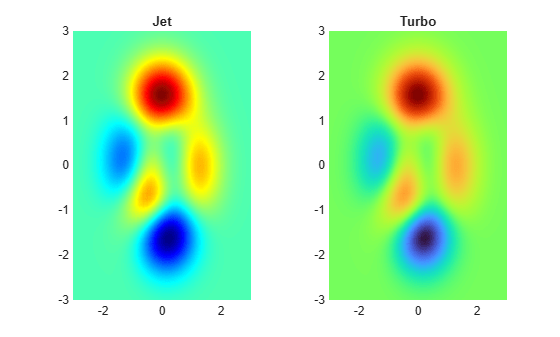## Input Arguments

collapse all

Number of colors, specified as a scalar integer value. The default value of `m` is equal to the length of the colormap for the current figure. If no figure exists, the default value is 256.

Data Types: `single` | `double`

## Version History

Introduced in R2020b